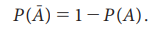Home | | Statistics 11th std | Axioms of Probability

# Axioms of Probability

i. Axiomatic approach to probability ii. Basic Theorems of Probability

Axioms of Probability

Andrey Nikolaevich Kolmogorov (1903ŌĆō1987) was a 20th-century Soviet mathematician who made significant contributions to the mathematics of probability theory, topology, intuitionistic logic, turbulence, classical ... Wikipedia

A.N. Kolmogorov proposed the axiomatic approach to probability in 1933. An axiom is a simple, indisputable statement, which is proposed without proof. New results can be found using axioms, which later become as theorems.## Axiomatic approach to probability

Let S be the sample space of a random experiment. If a number P(A) assigned to each event AŌłłS satisfies the following axioms, then P(A) is called the probability of A.

Axiom-1 : P (A) Ōēź 0

Axiom-2 : P (S) = 1

Axiom-3 : If {A1,A2,ŌĆ”} is a sequence of mutually exclusive events i.e., Ai Ōł® Aj  = ŽĢ

when i ŌēĀ j, thenAxiom-3 also holds for a set of finite number of mutually exclusive events. If A1, A2,ŌĆ”, An are mutually exclusive events in S and n is a finite positive integer, then

P(A1 Ōł¬ A2 ŌĆ” An) = P(A1) + P(A2) + ŌĆ” + P(An).

It may be noted that the previous two approaches to probability satisfy all the above three axioms.

## Basic Theorems of Probability

Theorem 8.1: The probability of impossible event is 0 i.e., P(ŽĢ) = 0.

Proof: Let A1 = S and A2 = ŽĢ. Then, A1 and A2 are mutually exclusive.Theorem 8.2: If S is the sample space and A is any event of the experiment, then### Proof:Theorem 8.3: If A and B are two events in an experiment such that A ŌŖéB, then P(B-A) = P(B) ŌĆō P(A).

### Proof:

It is given that A ŌŖé B.

The event B can be expressed as

B = A Ōł¬ (B-A) (see Figure 8.6)

Since A Ōł®(B-A) = Žå,

P(B) = P(A Ōł¬ (B-A))

Hence, by Axiom-3,

=> P(B) = P(A) + P(B-A)

Therefore, P(B-A) = P(B) ŌĆō P(A).Corollary: If A ŌŖéB, then P(A)Ōēż P(B).

### Proof:

Since, by Axiom-1, P(BŌĆōA) Ōēź 0, it follows that

P(B) ŌĆō P(A) Ōēź 0

P(B) Ōēź P(A)

=> P(A) Ōēż P(B).

### Example 8.8

In the experiment of tossing an unbiased coin (or synonymously balanced or fair coin), the sample space is S = {H, T}. What is the probability of getting head or tail?

### Solution :

If the events A1 and A2 are defined as A1 = {H} and A2 = {T}, then S = A1 ,A2.  Here, the events A1 and A2 are mutually exclusive, because they cannot occur together. Hence, by using Axiom-3, it can be written as

P(S) = P(A1)+P(A2)

Since the number of elementary events in S in favour of the occurrence of A1 and A2 is one each, they have equal chances to occur. Hence, P(A1) = P(A2). Substituting this, it follows thatThus, a coin is called unbiased, if the probability of getting head is equal to that of getting tail.

Aliter: (Applying Classical approach)### Example 8.9

Ammu has five toys which are identical and one of them is underweight. Her sister, Harini, chooses one of these toys at random. Find the probability for Harini to choose an underweight toy?### Solution :

It is seen from fig. 8.7, the sample space is S = {a1, a2, a3, a4, a5}.Define the events A1, A2, A3, A4 and A5 as

A : Harini chooses the underweight toyTherefore, the probability for Harini to choose an underweight toy is 1/5.

### Example 8.10

A box contains 3 red and 4 blue socks. Find the probability of choosing two socks of same colour.### Solution :

From fig. 8.8, total number of socks = 3 + 4 = 7

If two socks are drawn at random, then

No. of ways of selecting 2 socks = 7C2 = 21then A1 Ōł¬ A2 represents the event of selecting 2 socks of same colour. Since the occurrence of one event excludes the occurrence of the other, these two events are mutually exclusive. Then, by Axiom-3,Thus, the probability of selecting two socks of same colour is 3/7.

### Example 8.11

Angel selects three cards at random from a pack of 52 cards. Find the probability of drawing:

ii. one spade and two knave cards

iii. one spade, one knave and one heart cards.

### Solution:

Total no. of ways of drawing 3 cards = n(S) = 52 C3 = 22100

(a) Let A1 = drawing 3 spade cards.

Since there are 13 Spades cards in a pack of cards,

No. of ways of drawing 3 spade cards = n(A1) = 13 C3 = 286

Therefore, P(A1) =(b) Let A2 = drawing one spade and two knave cards

No. of ways of drawing one spade card = 13C1 = 13

No. of ways of drawing two knave cards = 13C2= 78

Since drawing a spade and 2 knaves should occur together,

No. of ways drawing one spade and two knave cards = n(A2) = 13├Ś78 =1014(c) Let A3 = drawing one spade, one knave and one heart cards

No. of ways of drawing one spade, one knave and one heart cards is

n(A3) = 13C1├Ś 13C1 ├Ś 13C1 = 13 ├Ś 13 ├Ś 13Tags : Theorems, Proof, Solved Example Problems , 11th Statistics : Chapter 8 : Elementary Probability Theory
Study Material, Lecturing Notes, Assignment, Reference, Wiki description explanation, brief detail
11th Statistics : Chapter 8 : Elementary Probability Theory : Axioms of Probability | Theorems, Proof, Solved Example Problems